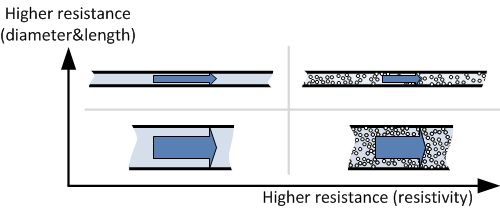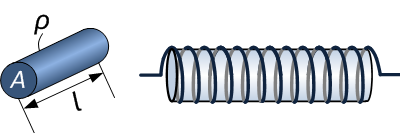# Electrical Resistivity

## What Is Electrical Resistivity?

Electrical resistivity is a measure of a material’s property to oppose the flow of electric current. This is expressed in Ohm-meters (Ω⋅m). The symbol of resistivity is usually the Greek letter ρ (rho). A high resistivity means that a material does not conduct electric charge well.

Electrical resistivity is defined as the relation between the electric field inside a material, and the electric current through it as a consequence:

$$\rho = \frac{E}{J}$$

in which ρ is the resistivity of the material (Ω⋅m),

E is the magnitude of the electrical field in the material (V/m),

J is the magnitude of the electric current density in the material (A/m2)

If the electric field (E) through a material is very large and the flow of current (J) is very small, it means that the material has a high resistivity.

Electrical conductivity is the inversion of resistivity and is a measure of how well a material conducts electric current:

$$\sigma = \frac{1}{\rho} = \frac{J}{E}$$

in which σ is the conductivity of the material expressed in siemens per meter (S/m). In electrical engineering, κ (kappa) is used often instead of σ.

## Electrical Resistance

Electrical resistance is expressed in ohms, and is not the same as resistivity. While resistivity is a material property, resistance is the property of an object. The electrical resistance of a resistor is determined by the combination of the shape and the resistivity of the material. For example, a wirewound resistor with a long, thin wire has a higher resistance than with a shorter and thicker wire. A wirewound resistor made from a material with high resistivity has a higher resistance value then one with a low resistivity. An analogy with a hydraulic system can be made, where water is pumped through a pipe. The longer and thinner the pipe, the higher the resistance will be. A pipe full with sand will resist the flow of water more than a pipe without sand (resistivity property).Hydraulic analogy of electrical resistance

### Wire resistance

The resistance value of a wire depends on three parameters: resistivity, length and diameter. The formula to calculate wire resistance is as follows:

$$R = \rho (\frac{l}{A})$$

in which R is the resistance (Ω),

ρ is the resistivity of the material (Ω⋅m),

l is the length of the material (m),

A is the cross-sectional area of the material (m2)The resistance value of a wire is dependent on three parameters; its resistivity, cross-sectional area and length.

As an example, consider a wirewound resistor with a wire of Nichrome with a resistivity of 1.10×10−6 Ω⋅m. The wire has a length of 1500 mm (1.5 m) and an area of 0.5 mm2 (0.5×10−6 m2). With these three parameters the resistance value is calculated:

$$R = \rho (\frac{l}{A}) = 1.1⋅10^{-6} ⋅ \frac{1.5}{0.5⋅10^{-6}} = 3.3 \Omega$$

Nichrome and Constantan are often used as resistance wire. Look in the table for material resistivity for commonly used materials.

### Sheet resistance

The resistance value for a sheet is calculated the exact same way as for wire resistance. The cross-sectional area can be written as the product of w and t:

$$R = \rho (\frac{l}{A}) = \rho (\frac{l}{wt})$$

For some applications like thin films, the ratio between resistivity and film thickness is called sheet resistance Rs:

$$R = \rho (\frac{l}{wt}) = R_s (\frac{l}{w})$$

in which Rs is in ohms/square (Ω/□). The film thickness needs to be uniform for this calculation.

Often resistor manufacturers increase resistance by cutting a pattern in the film to increase the path of the electric current.Electrical resistance of a sheet depends on length, width, film thickness and resistivity. The resistance can be increased by cutting a pattern in the sheet.

## Resistive Properties of Materials

The resistivity of a material is dependent on the temperature and is normally given for room temperature (20°C). The change in resistivity as a result of temperature change is described by the temperature coefficient. For example, thermistors make use of this property to measure temperature. On the other hand, in precision electronics this is usually an unwanted effect. Metal foil resistors have excellent properties for temperature stability. This is achieved not only by the low resistivity of the material, but also by the mechanical design of the component.

Many different materials and alloys are used for resistors. Nichrome, an alloy of nickel and chromium, is often used as resistor wire material because of its high resistivity, and because it doesn’t oxidize at high temperatures. A disadvantage is that solder doesn’t adhere to it. Constantan, another popular material, is easily soldered and has a low temperature coefficient.

 Material ρ (Ω⋅m) at 20°C σ (S/m) at 20°C Temperature coefficient (1/°C) x10-3 Silver 1.59×10−8 6.30×107 3.8 Copper 1.68×10−8 5.96×107 3.9 Gold 2.44×10−8 4.10×107 3.4 Aluminum 2.82×10−8 3.5×107 3.9 Tungsten 5.60×10−8 1.79×107 4.5 Zinc 5.90×10−8 1.69×107 3.7 Nickel 6.99×10−8 1.43×107 6 Lithium 9.28×10−8 1.08×107 6 Iron 1.0×10−7 1.00×107 5 Platinum 1.06×10−7 9.43×106 3.9 Tin 1.09×10−7 9.17×106 4.5 Lead 2.2×10−7 4.55×106 3.9 Manganin 4.82×10−7 2.07×106 0.002 Constantan 4.9×10−7 2.04×106 0.008 Mercury 9.8×10−7 1.02×106 0.9 Nichrome 1.10×10−6 9.09×105 0.4 Carbon (amorphous) 5×10−4 to 8×10−4 1.25 to 2×103 -0.5